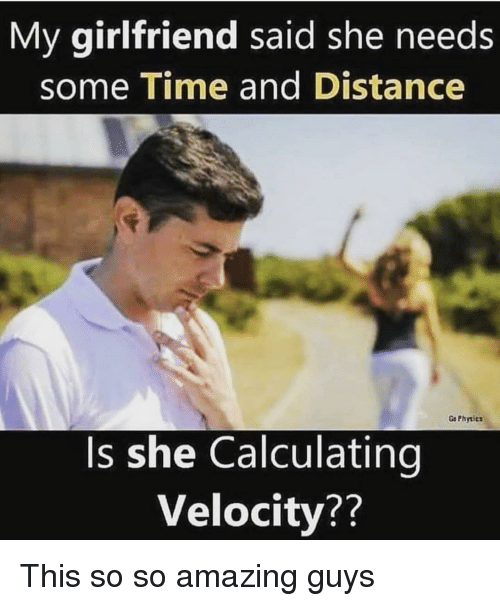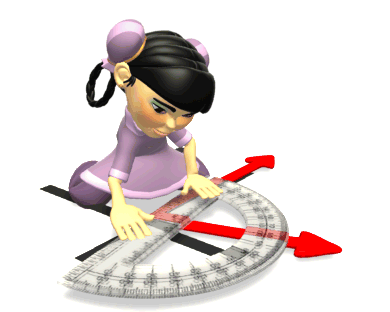Invisible Flame

Review

Drag and drop the runners on the track below to show:

a) constant velocity b) acceleration

Video Instruction

showmethephysics.com

E. Solving Problems using the motion equations

 Ex 3) Starting from rest, an object accelerates at a rate of 12 m/s2. What is the velocity of the object at the end of 3.0 seconds?Motion Map

1st sec / 2nd sec / 3rd sec12 m/s  / 24 m/s / 36 m/sEx 3) Starting from rest, an object accelerates at a rate of 12 m/s2. What is the velocity of the object at the end of 3 seconds?

Vi = 0 (Starting from rest)

a = 12 m/s2

Vf = ?

t = 3.0 seconds

a = (Vf - Vi)/t

12 m/s2 = (Vf - 0)/3.0 secs

(Cross Multiply and Solve)

Vf = 36 m/sEx 4) An object at 100. m/s accelerates at a rate of 120. m/s2. What will the velocity of this object be after .10 seconds?

Underline Info

Ex 4) An object at 100. m/s accelerates at a rate of 120. m/s2. What will the velocity of this object be after .100 seconds?

a = (Vf - Vi)/t

120. m/s= (Vf - 100. m/s)/.100 s

• Cross Multiply

12.0 = (Vf - 100.)

Vf = 112 m/s

3 ways to have non-zero acceleration

(to change velocity):

Speed Up• Slow Down• Change DirectionF. Motion Terms and Direction

 A whole branch of mathematics is required to deal with numbers that have direction   TrigonometryThis is why it is important to know which motion information has direction

Vector - a quantity that includes magnitude (a number) & directionex) displacement, acceleration, velocity

Scalar - a quantity that includes magnitude (a number) only

ex. time, distance, speed